## Modelling in Fashion

I have been trying to get more familiar with image related problems, having heard interesting stories about the powerful applications and dangers of applying data science to images. I decided an interesting place to start would be to get familiar with existing frameworks and come up with a novel application.

The idea in question is to explore how fashion brands selectively choose certain types of people to showcase their products. I then want to use the learnings from this to build an application that can take an image of anyone and decide which brands they are most alligned with.

## Sourcing the Images

The data to start using would be forward facing, high definition, colour images of people with uncovered faces. I decide to scrape a list of images for male and female products that fit this criteria for some popular brands: Tom Ford, Uniqlo and Urban Outfitters. These raw images are then stored locally and manually checked to see if they are all suitable for analysis.

The two problems I will face when carrying out this project:

1. Face Detection
Images taken in the real world are not homogenous, they come in different sizes, lightings, quality and where the main subjects appear in different places. Face Detection is the ability for a machine to be able to identify a face in any context it can appear in.
2. Facial Recognition
In many applications of image-related solutions, the purpose is to match a face against another. I'm talking about Horatio hoping to find the killer's face in his database of criminal mugshots, or perhaps in a more ominous tone, automatically issuing fines to jaywalkers using CCTV pictures. Facial recognition is the ability to be able to compare one face to another and determine if the face belongs to the same person.

## Haar Cascade Classification | Face Detection

All of the images I have downloaded contain non-facial features so I will need to extract just the faces as the initial treatment to apply; this falls under the first problem mentioned above.

In my first attempt, I use a well-known introductory method called Haar Cascade Classifiers based on a concept introduced in 2001. The key concepts to understand in this framework are below.

##### Haar-Like Features

We create features from each image by looking at the pixel intensities for different rectangular regions of the image. For each region of the image, we overlay the shapes below, called a Haar feature, and minus the sum of pixel intensities of the white sub-regions from the black sub-regions.Haar feature formula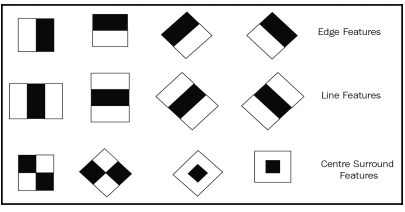What a Haar feature looks like

The number of features to create can easily balloon with the size of each image and the size of the regions. For example, For example, an image with dimensions (24,24) pixels would generate over 160,000 features, making real time face detection impossible. In practise, there is significant optimisations such:

- calculations of features made quicker through the use of integral images and,
- the number of features generated is less than the upper bound due to the cascading nature of the classifier.

##### Integral Image

If we can consider every input image as a 2-dimensional array of pixel intensities, we create a haar-like feature by calculating the difference of the sums of a few rectangular sub-arrays. This can become computationally expensive, so the 2001 paper introduced the idea of using an Integral Image as a intermediate step to perform these calculations.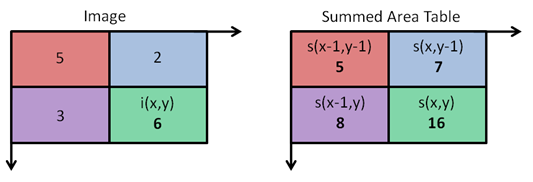Computing an integral image

This is an example of how to create an integral image, or a summed area table. The value at any point (x,y) is the sum of all of the values above and to the left of that point inclusive.whereis the pixel intensity at a point (x,y).

We create the integral image from the original image efficiently (in a single pass) of the image by usingOnce the image has been created, we can calculate the sum of of all pixel intensities in a region bounded by A=(x0, y0), B=(x1, y0), C=(x0, y1) and D=(x1, y1) using the formulaas shown by this example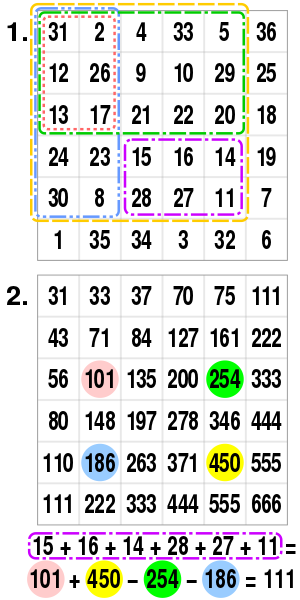Integral image example

This means that with this method, we can build the integral image in O(n) and we can calculate the sums of regions in O(1). This is much quicker than using the naive method of summing individual pixels which would have complexity O(m2) for a region of m x m.

##### Sliding Window

I have been using the term 'regions' frequently without defining what a region is in the context of face detection. A region, as you may have guessed, is some rectangular window of the image. For any image, we create regions to generate features from by using the concept of a sliding window. This is essentially a sequence of regions that moves across the image pixel by pixel as shown by this diagram.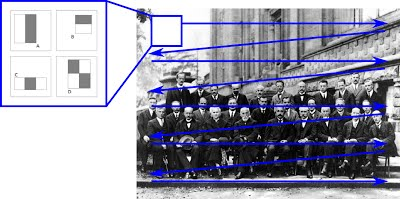Sliding window

The approach above describes an efficient method of generating features. However, a large problem that remains is that in any image there are many regions each of which will generate many features. Given that most of an image is not a face, most of the processing involved to generate a prediction will be redundant. The idea of a cascading classifier solves this problem.

A cascade classifier consists of multiple stages of filters, as shown in the figure below. Each time the sliding window shifts, the new region within the sliding window will go through the cascade classifier stage-by-stage. If the input region fails to pass the threshold of a stage, the cascade classifier will immediately reject the region as a face. If a region passes all stages successfully, it will be classified as a candidate of face. This process is applied for many regions of different sizes of windows.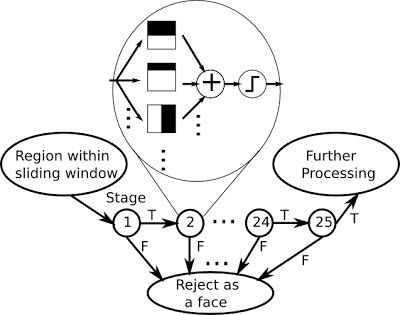Classifier process

#### Extracting Faces

The python library OpenCV contains an implementation of this cascade classification method and a rudimentary prediction on a directory of images containing faces can be made in under 40 lines. Begin by importing the necessary modules and creating variables to the relevant folders and files.

```        import cv2
import sys
import os

RAW_IMAGE_DIRECTORY = os.path.dirname(os.path.dirname(os.path.abspath(__file__))) + '/data/images/raw/'
PROCESSED_IMAGE_DIRECTORY = os.path.dirname(os.path.dirname(os.path.abspath(__file__))) + '/data/images/processed/'
```

Here the HAAR_CASCADE_FILE contains the weights to the trained classifier. Then create the classifier object with

```        face_cascade = cv2.CascadeClassifier(HAAR_CASCADE_FILE)
```

Then we can loop through the files, read them into a python object and predict the bounding region for all found faces. These regions are then cropped from the image and saved into a new directory.

```        for filename in os.listdir(RAW_IMAGE_DIRECTORY):
if filename == '.DS_Store':
continue

gray = cv2.cvtColor(image, cv2.COLOR_BGR2GRAY)

# Detect faces in the image
gray,
scaleFactor=1.1,
minNeighbors=5,
minSize=(30, 30),
)

# Draw a rectangle around the faces
# Extract faces
for i, (x, y, w, h) in enumerate(faces):
cv2.imwrite(PROCESSED_IMAGE_DIRECTORY+filename+'_'+str(i)+'.jpeg', image[y:y+h, x:x+w])
cv2.rectangle(image, (x, y), (x+w, y+h), (0, 255, 0), 2)

cv2.imwrite(PROCESSED_IMAGE_DIRECTORY+filename+'.jpeg', image)
```

Here are some examples of the output of this script.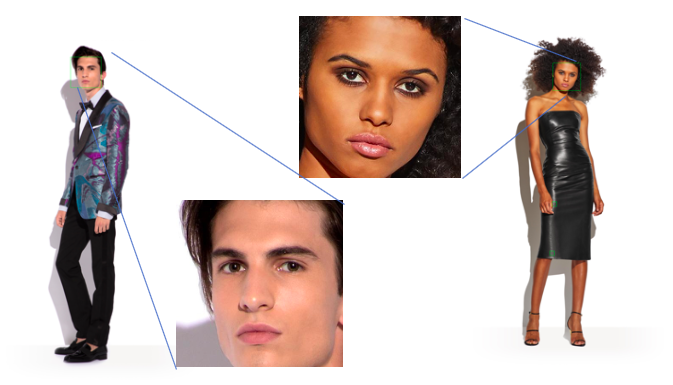Extracted faces

In total, out of a total of 90 images, this algorithm correctly identified and extracted 74 images (82%), 10 (11%) images had no face identified, and 6 (7%) had an incorrect face identified. Looking closer at the images where this method performed poorly, it is clear that this is a problem of skin colour. Those with darker tones had a correct face identification rate of 27%.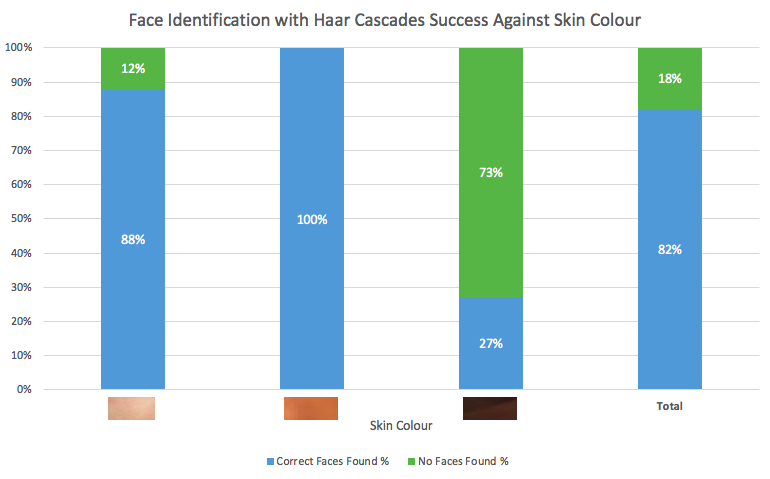Racially biased classifier

Thinking about how the Haar features area created, i.e. using the difference of sums of pixel intensities, it is a an unsurprising result for faces where the captured image shows little variation shades.

This is a well known problem for many face identification problems and poses serious ethical concerns that are necessary to address when building applications that utilise these methods.